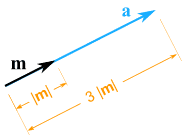Definition of

# ScalarA number on its own (used when dealing with vectors or matrices).

Example: we can multiply the vector (5,2) by the scalar 3 to get a new vector (15,6)
3 × (5,2) = (3×5,3×2) = (15,6)

See: Vector